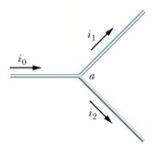# Fundamentals of Physics Study Set 2

Physics & Astronomy

## Quiz 26 :Current and ResistanceLooking for Introductory Physics Homework Help?

## Quiz 26 :Current and Resistance

Showing 1 - 20 of 54A car battery is rated at 80 Ah.An ampere-hour is a unit of:
Free
Multiple Choice

DCurrent has units:
Free
Multiple Choice

BCurrent has units:
Free
Multiple Choice

BCurrent is a measure of:
Multiple ChoiceA 60-watt light bulb carries a current of 0.5 ampere.The total charge passing through it in one hour is:
Multiple ChoiceA 10-ohm resistor has a constant current.If 1200 C of charge flow through it in 4 minutes what is the value of the current?
Multiple ChoiceCurrent in a conductor is normally due to:
Multiple ChoiceThis figure shows a junction.What is true of the currents?Multiple ChoiceIn schematic diagrams, currents are indicated using arrows.What do the arrows indicate?
Multiple ChoiceConduction electrons move to the right in a certain wire.This indicates that:
Multiple ChoiceIf is the current density and is a vector element of area then the integral over an area represents:
Multiple ChoiceTwo wires made of different materials have the same uniform current density.They carry the same current only if:
Multiple ChoiceA wire with a length of 150 m and a radius of 0.15 mm carries a current with a uniform current density of 2.8 107 A/m2.The current is:
Multiple ChoiceIn a conductor carrying a current we expect the electron drift speed to be:
Multiple ChoiceThe current is zero in a conductor when no potential difference is applied because:
Multiple ChoiceThe current density is the same in two wires.Wire A has twice the free electron concentration of wire B.The drift speed of electrons in A is:
Multiple ChoiceCopper contains 8.4 1028 free electrons per cubic meter.A copper wire of cross-sectional area 7.4 10-7 m2 carries a current of 1 A.The electron drift speed is approximately:
Multiple ChoiceThe units of resistivity are:
Multiple Choice